• 2018-11-01 20:30:35
#include<stdio.h>
int main()
{
double x,x1,x2,w;
scanf("%lf",&x);
x1=x;
x2=(2.0*x1+x/(x1*x1))/3.0;
w=(x2-x1)/x1;
if(w<0)
w=w*-1;
while(w>=1e-6)
{
x1=x2;
x2=(2*x1+x/(x1*x1))/3.0;
w=(x2-x1)/x1;
if(w<0)
w=w*-1;

}
printf("%f\n",x2);
}


更多相关内容
• 问题描述： 编译环境：vc++6.0 代码： #include #include int main() { double num, x0, x1; printf("请输入x的值\n"); scanf("%lf", &num);... } printf("x的立方根为%lf\n", x1); return 0; } 结果：

问题描述：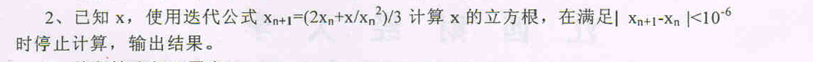编译环境：vc++6.0

代码：

#include <stdio.h>
#include <math.h>
int main()
{
double num, x0, x1;
printf("请输入x的值\n");
scanf("%lf", &num);
x0 = num;
x1 = (2*x0+num/(x0*x0))/3;
while (fabs(x1-x0)>10e-6)
{
x0 = x1;
x1 = (2 * x0 + num / (x0 * x0)) / 3;
}
printf("x的立方根为%lf\n", x1);
return 0;
}


结果：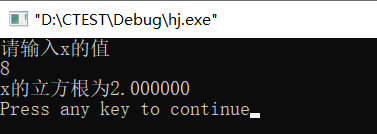展开全文迭代
• 一个求实数立方根C语言函数（牛顿迭代法） 已知利用牛顿迭代法方程F（x）=0的解的公式为X[n+1] = X[n] - F(X[n])/F'(X[n]),其中x[n]为第n次利用此公式求得值。 假如函数F(X) = X*X*X + a, 则根据牛顿迭代...

已知利用牛顿迭代法求方程F（x）=0的解的公式为X[n+1] = X[n] - F(X[n])/F'(X[n]),其中x[n]为第n次利用此公式求得值。

假如函数F(X) = X*X*X + a, 则根据牛顿迭代法第n+1次求方程F（x） = 0的解为X[n+1]， 且X[n+1] = X[n] -  (X[n]*X[n]*X[n]+a)/3*X[n]*X[n]。

利用C语言实现求一个实数立方根的代码如下：

 // -- cuberoot.c -- 求一个实数的立方根 typedef enum Boolean{FALSE, TRUE}Boolean;   /*      函数： double CubeRoot(double)      功能： 求解一个数的立方根       算法： 牛顿法       使用： 输入一个实数，输出输入实数的立方根  */ double CubeRoot( const  double stuff) {      const  double  precision = 0.00001;  /* 精度 */      double nextX, currentX;      double squareX;      /* 当前值 的平方 */      double cubeX;        /* 下个解 的立方 */      Boolean flag;            currentX = stuff;   /* 初始化当前的值为X */            if  (stuff == 0)      {          return  stuff;      }      else      {          do          {              squareX = currentX * currentX;              nextX = (2*currentX + stuff/squareX) / 3;        /* 牛顿迭代公式 */              cubeX = nextX*nextX*nextX;              if  ( (cubeX - stuff < precision) && (cubeX - stuff > -precision))              {                  flag = TRUE;              }                 else              {                  flag = FALSE;                  currentX = nextX;              }          } while (flag == FALSE);      }            return  nextX; }
展开全文• 思路三：牛顿迭代法 首先要先了解牛顿迭代法是什么： 假如输入 a ，数 a 的立方根，那么 x^3-a=0 这个方程的解就是我们所的。 而方程 f(x) 的牛顿迭代格式为： 所以，所方程的牛顿迭代公式为： 了解了牛顿...

也不开学，只能自己找点事情干了。O(∩_∩)O

虽然周六还有一场考试，周五还有一个Presentation，周四还有一下午的实验，周三还有一上午的课，可是，我 都 不 想 干 ~

又不会做什么大事情，只能做点小题目来觉得自己没有闲着，干了大事情，难搞。/(ㄒoㄒ)/~~

牛客做题的第一天，只写了两道题，还看了别人的思路，脑子不在状态，写不出来自己的代码/(ㄒoㄒ)/~~

【前面这一大段废话是两周前写的，现在才来干正事，我真是个废物】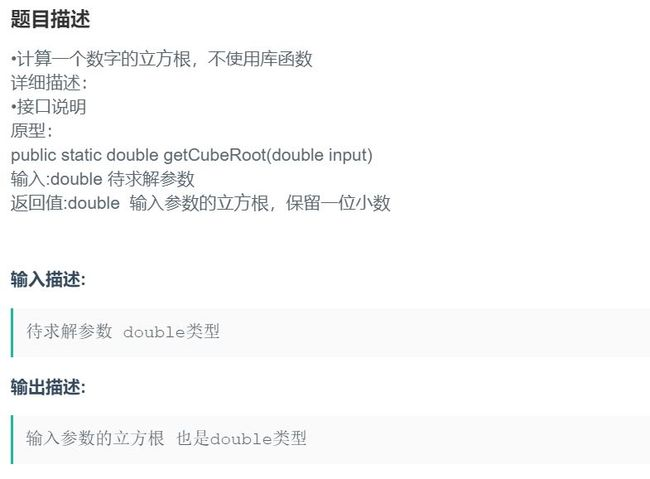思路一：库函数

函数 pow(x,y) 是计算 x 的 y 次方，所以，令 y=1/3 即可求数 x 的立方根。

思路二：二分法

public static double getCubeRoot(double input)

{

if(input==0||input==1||input==-1)

return input;

else

{

double min = 0;

double max = input;

double mid = 0;

// 注意，这里的精度要提高一点，否则某些测试用例无法通过

while ((max - min) > 0.001)

{

mid = (max + min) / 2;

if (mid * mid * mid > input)

max = mid;

else if (mid * mid * mid < input)

min = mid;

else

return mid;

}

return max;//这种情况是：在满足精确度的条件下无精确值，选择max作为近似值来返回。

//同样也可以选择min作为近似值来返回

}

}

我觉着这个代码还是很简单的，主要是理解二分法的思想：

在这里，是先把立方根的范围锁定在0-x(x是输入的值)。

mid=(min+max)/2;

然后让 mid 连续三次乘，结果为 y

然后比较 y 与 input 的关系。

如果y 大于 input 时，max=mid，范围缩小了一半；

如果y 小于 input 时，min=mid，范围缩也小了一半；

如果y 等于 input 时，代表 mid 就是数 input 的立方根，可直接返回。

思路三：牛顿迭代法

首先要先了解牛顿迭代法是什么：

假如输入 a ，求数 a 的立方根，那么 x^3-a=0 这个方程的解就是我们所求的。

而方程 f(x) 的牛顿迭代格式为：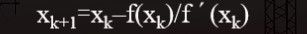所以，所求方程的牛顿迭代公式为：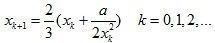了解了牛顿迭代法的内容，我们就可以来写代码了。

public static void main(String[] args) {

double x,y,x1,x2;

Scanner in = new Scanner(System.in);

x = in.nextDouble();

System.out.println("请手动输入一个初始近似值");

x1 = in.nextDouble();

in.close();

x2= (x/x1/x1+2*x1)/3; //牛顿迭代公式的第一次使用

y = fun(x2,x);

System.out.printf("%.1f",y);

}

public static double fun(double x2,double x)

{

if(Math.abs(x2*x2*x2-x)<0.0000001) //递归的结束条件

return x2;

else

return fun((x/x2/x2+2*x2)/3,x);//牛顿迭代公式的递归使用

}

OK，终于把这个在草稿箱里躺了两周的文章写完了。睡觉咯，晚安。

展开全文• #include<stdio.h> int main() { double x,x1,x2,w; scanf("%lf",&x); x1=x; x2=(2.0*x1+x/(x1*x1))/3.0; w=(x2-x1)/x1; if(w<0) w=w*-1; while(w>...
• 在这里，我们将看到如何获得数字的立方根。假设一个数字为27，则该数字的立方根为3。为解决此问题，我们将定义自己的逻辑，而无需使用某些库函数。我们将使用二进制搜索方法。我们必须按照以下步骤解决此问题。假设...
• 计算一个数字的立方根方法：使用对数和指数组合的方法表示立方根
• ## 二分法求立方根

千次阅读 2020-06-04 19:14:24
function lfg(x){ if(x==0){ return 0; } let min = 0,max = x; if(x<0){ min = x; max = 0; } while(min < max){ let mid = (min + max) / 2; //二分法 let tmp = mid*mid*mid;... x) {算法 javascript
• #include "iostream" using namespace std; int main() { double x1,x,y,z; cin>>x1; for(x=x1,y=2*x/3+x1/(3*x*x),z=x; y-z<..."立方根为："<<x<<endl; system("pause"); r.html
• 满意答案waoc0yjg82013.12.30采纳率：58%等级：12已帮助：6598人考虑数列a...0,a为你想求立方根的数。1.a(n)≥a的立方根对任意n≥2成立。证明：a(n+1)={2a(n)+a/{[a(n)]^2}}/3={a(n)+a(n)+a/{[a(n)]^2}}/3≥a的立方...
• 介绍使用牛顿迭代法求立方根x3{\sqrt{x}}3x​的C语言实现和公式的推导。 代码 float CubeRoot(float num) { float x = num; float error = 1e-5; while (fabs(num - (x * x * x)) >= error) { x = (2 *...算法
• 平方根的方法，称为笔算开平方法，用这个方法可以出任何正数的算术平方根，它的计算步骤如下： 1．将被开方数的整数部分从个位起向左每隔两位划为一段，用撇号分开(竖式中的11'56)，分成几段，表示所平方根...
•算法
• //其作用是计算x的y次方 用c语言利用泰勒公式sin(x)的值 要求最后一项的值小于 pow(10,-5) 最好把。 //欢迎在线讨论#include #include long F(int n) { long s=1; int i; for(i. i+=2) { if((i+1)%4==0) sum-=pow...
• //计算一个数字的立方根，不使用库函数 //详细描述： //接口说明 //原型： //public static double getCubeRoot(double input) //输入:double 待求解参数 //返回值:double 输入参数的立方根数据结构
• 假设要求立方根的数为num 则我们就是要求x^3=num的解 即 x^3-num=0的解 构造函数f(x)=x^3-num 在函数上任取一点(x1,y1) 此时y1= f(x1) =x1^3 - num 而这一点的导数为y1' = f'(x1) = 3x1^2 于是可以列出此处...算法 c++
• #include&lt;stdio.h&gt;#include&lt;stdlib.h&gt;#include&lt;time.h&gt;main(){ srand(time(NULL)); int a,i,j,k,t; for(i=0;i&lt;10;i++) { a[i]=rand()%100;...,a[j]...
• (教案3)6.2立方根共享者：ml共享时间：2015/6/25下载：次资源类别：教案资源属性：同步课程适用地区：北京关键字：立方根立方根》课程目标一、知识与技能目标1．了解立方根的概念，能够用根号表示一个数的立方根．...
• 给一个浮点数num, 如何立方根ans？首先 0 < |ans| < num，对于浮点数区间的海量数据，若采用加法枚举判断，那绝对把CPU能累死。计算精度越高，时间复杂度越高。 上述方法，只是简单的加法性线性探测。 ...
• 查找数字的立方根 这可以使用三个方法来实现 1.猜猜并检查 检查每个猜测以确认它是正确的多维数据集根。 此方法只能用于找到理想立方体的立方体根。 2.近似值 这给出了一个足够好的解决方案，一个足够接近多维数据集...Python
• } /** * 1/3乗をして立方根める * @param[in] x 実数 * @return x の立方根 */ double cbrt_simple(double x) { return pow(x, 1.0 / 3.0); } /** * ニュートン法で立方根を近似する * @param[in] a 実数 * @...
• 它要求立方根精确到小数点后一位，这里要注意。 注意输出的话，因为有要求，所以不能直接用cout,而是用printf(".1lf",mid) #include<iostream> using namespace std; int main(){ double a; cin>>a...算法 二分法 c++
• 有三个担心，我有。你在你的表达式中的一部分利用Math.Pow()，但是，你会想在谈话过程中，后来给出的表达式的第一部分使用Math.Sqrt()。其次，与表达中的括号分组导致表达的错误评价的问题第三，你将需要你没有一个...
• //do while #include <stdio.h> #include <math.h> int main(){ float x0, x1; float a; x1 = 1; printf("请输入a(不为0):"); scanf("%f", &a); // do{ x0 = x1; ... .开发语言 后端
• •计算一个数字的立方根，不使用库函数 详细描述： •接口说明 原型： public static double getCubeRoot(double input) 输入:double 待求解参数 返回值:double 输入参数的立方根，保留一位小数 输入格式 待求解参数...
• 介绍使用魔数0x2a517d47快速求立方根x3{\sqrt{x}}3x​的C语言实现和公式的推导。 代码 float MagicCubeRoot(float x) { float xthird = 0.333f * x; int i = *(int*)&x; i = (0x2a517d47 + (0.333f * i));...算法
• 要求：计算一个数字的立方根，不使用库函数 输入:double 待求解参数 返回值:double 输入参数的立方根，保留一位小数 思路：二分法 import java.util.*; public class Main{ public static double getCubeRoot...java
• 前言 求解立方根. 原题信息如下所示: 计算一个数字的立方根，不使用库函数 详细描述： 接口说明 原型： public static double getCubeRoot(double input) 输入:double 待求解参数...求立方根. 工具法. 我们可以使用M算法 pow
• 牛顿迭代公式平方根迭代公式：a(n+1)=( a(n) + num/a(n)... 立方根迭代公式：a(n+1)=( 2a(n) + num/( (a(n))^2 ) )/3，a(0) 初始化为1；程序计算过程#include #include #define E 0.0001 using namespace std;double ge...